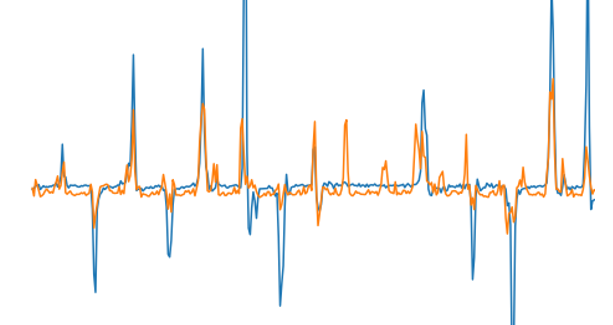# Constant offset with NN regression

I’m using a simple 4-layer feedforward network to predict velocities, which can be positive and negative. The first 3 layers use ReLU and the final layer is linear to be able to output negative and positive values. I’m using Adam with MSE loss and I stop training when validation loss stops improving.

The network trains and converges to a solution relatively fast, however, the final network predictions often have a constant offset/bias relative to the true data. I would expect the MSE loss to minimize this constant offset. Could this be related to learning rate, or should I try a different loss that has a larger penalty on small offsets?

Here is an example output on test-data, where blue is the true velocity and orange is the NN predicted velocity. The orange has a constant negative bias.